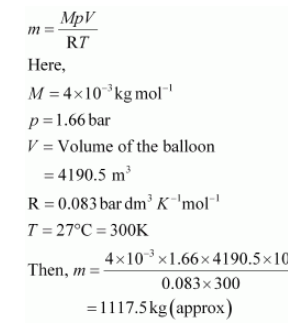# Pay load is defined as the difference between the mass of displaced air and the mass of the balloon.`
Question:

Pay load is defined as the difference between the mass of displaced air and the mass of the balloon. Calculate the pay load when a balloon of radius $10 \mathrm{~m}$, mass $100 \mathrm{~kg}$ is filled with helium at $1.66$ bar at $27^{\circ} \mathrm{C}$. (Density of air $=1.2 \mathrm{~kg} \mathrm{~m}^{-3}$ and $\mathrm{R}=0.083$ bar $\mathrm{dm}^{3} \mathrm{~K}^{-1} \mathrm{~mol}^{-1}$ ).

Solution:

Given,

Radius of the balloon, r = 10 m

$\therefore$ Volume of the balloon $=\frac{4}{3} \pi r^{3}$

$=\frac{4}{3} \times \frac{22}{7} \times 10^{3}$

$=4190.5 \mathrm{~m}^{3}($ approx $)$

Thus, the volume of the displaced air is $4190.5 \mathrm{~m}^{3}$.

Given,

Density of air = 1.2 kg m–3

Then, mass of displaced air = 4190.5 × 1.2 kg

= 5028.6 kg

Now, mass of helium (m) inside the balloon is given by,Now, total mass of the balloon filled with helium = (100 + 1117.5) kg

= 1217.5 kg

Hence, pay load = (5028.6 – 1217.5) kg

= 3811.1 kg

Hence, the pay load of the balloon is 3811.1 kg.